Singmaster's conjecture ★★

Author(s): Singmaster

Conjecture   There is a finite upper bound on the multiplicities of entries in Pascal's triangle, other than the number.

The numberappears once in Pascal's triangle,appears twice,appears three times, and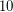appearstimes. There are infinite families of numbers known to appeartimes. The only number known to appeartimes is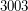. It is not known whether any number appears more thantimes. The conjectured upper bound could be; Singmaster thought it might beor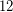. See Singmaster's conjecture.

Keywords: Pascal's triangle

Waring rank of determinant ★★

Author(s): Teitler

Question   What is the Waring rank of the determinant of ageneric matrix?

For simplicity say we work over the complex numbers. Thegeneric matrix is the matrix with entriesfor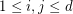. Its determinant is a homogeneous form of degree, in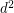variables. Ifis a homogeneous form of degree, a power sum expression foris an expression of the form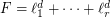, the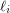(homogeneous) linear forms. The Waring rank ofis the least number of termsin any power sum expression for. For example, the expression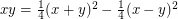means that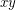has Waring rank(it can't be less than, as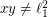).

Thegeneric determinant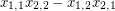(or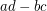) has Waring rank. The Waring rank of the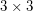generic determinant is at least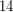and no more than, see for instance Lower bound for ranks of invariant forms, Example 4.1. The Waring rank of the permanent is also of interest. The comparison between the determinant and permanent is potentially relevant to Valiant's "VP versus VNP" problem.

Keywords: Waring rank, determinant

Monochromatic vertex colorings inherited from Perfect Matchings ★★★

Author(s):

Conjecture   For which values ofandare there bi-colored graphs onvertices anddifferent colors with the property that all themonochromatic colorings have unit weight, and every other coloring cancels out?

Keywords:

Cycle Double Covers Containing Predefined 2-Regular Subgraphs ★★★

Author(s): Arthur; Hoffmann-Ostenhof

Conjecture   Letbe a-connected cubic graph and letbe a-regular subgraph such that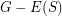is connected. Thenhas a cycle double cover which contains(i.e all cycles of).

Keywords:

Monochromatic reachability in arc-colored digraphs ★★★

Author(s): Sands; Sauer; Woodrow

Conjecture   For every, there exists an integersuch that ifis a digraph whose arcs are colored withcolors, thenhas aset which is the union ofstables sets so that every vertex has a monochromatic path to some vertex in.

Keywords:

3-Decomposition Conjecture ★★★

Author(s): Arthur; Hoffmann-Ostenhof

Conjecture   (3-Decomposition Conjecture) Every connected cubic graphhas a decomposition into a spanning tree, a family of cycles and a matching.

Keywords: cubic graph